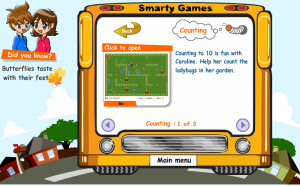# Online Counting GamesOnline Counting Games – Three free online counting games.  Great for preschool, kindergarten or any kid who is ready to learn to count.

Know number names and the count sequence.
CCSS.Math.Content.K.CC.A.1
Count to 100 by ones and by tens.
CCSS.Math.Content.K.CC.A.2
Count forward beginning from a given number within the known sequence (instead of having to begin at 1).
CCSS.Math.Content.K.CC.A.3
Write numbers from 0 to 20. Represent a number of objects with a written numeral 0-20 (with 0 representing a count of no objects).
Count to tell the number of objects.
CCSS.Math.Content.K.CC.B.4
Understand the relationship between numbers and quantities; connect counting to cardinality.
CCSS.Math.Content.K.CC.B.4.a
When counting objects, say the number names in the standard order, pairing each object with one and only one number name and each number name with one and only one object.
CCSS.Math.Content.K.CC.B.4.b
Understand that the last number name said tells the number of objects counted. The number of objects is the same regardless of their arrangement or the order in which they were counted.
CCSS.Math.Content.K.CC.B.4.c
Understand that each successive number name refers to a quantity that is one larger.
CCSS.Math.Content.K.CC.B.5
Count to answer “how many?” questions about as many as 20 things arranged in a line, a rectangular array, or a circle, or as many as 10 things in a scattered configuration; given a number from 1-20, count out that many objects.